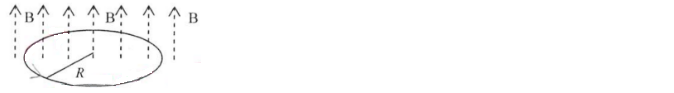# Problem: A positive charge q = 1.60 x 10 -19 C moves in a horizontal circle with a speed v = 3.65 x 107 m/s in a homogeneous and perpendicular magnetic field of 1.20 Tesla as shown. Calculate the centripetal force F on the charge.

###### Problem Details

A positive charge = 1.60 x 10 -19 C moves in a horizontal circle with a speed v = 3.65 x 107 m/s in a homogeneous and perpendicular magnetic field of 1.20 Tesla as shown. Calculate the centripetal force F on the charge.